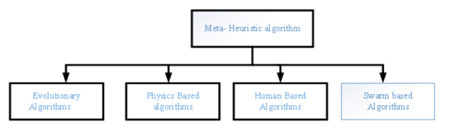• P-ISSN 0974-6846 E-ISSN 0974-5645# Indian Journal of Science and Technology

## Article• VIEWS 1512
• PDF 223

Indian Journal of Science and Technology

Year: 2021, Volume: 14, Issue: 28, Pages: 2380-2390

Original Article

## Mixed Method for Model Order Reduction Using Meta Heuristic Harris Hawk and Routh Hurwitz Array Technique

Received Date:10 June 2021, Accepted Date:05 July 2021, Published Date:28 August 2021

## Abstract

Abstract: A physical system is of higher-order and it is hectic for researchers to understand these systems in higher mathematical form. So, there is a requirement for systematic conversion of higher-order into a lower order. The lower order approximately gives the same result as that of the higher-order by preserving the important properties of higher-order. But the lower order retains some approximation error. Objective: The objective is to optimise the reduced-order by minimizing the integral square error between the higherorder system (HOS) and the lower-order system (LOS). Methodology: For the optimization process the novel harris hawk hunting behaviour is optimized. It is applied to find the unknown numerator by applying the novel algorithm. The denominator parameter is obtained by the Routh Hurwitz Array technique. Finding: The proposed technique is applied on a linear time-invariant single input single output system of higher-order which is randomly selected from the literature. To justify the proposed technique, the result obtained is compared with the result available in the literature. The comparison is based on the step response characteristics of the diminished order with original and result accessed from literature. The response indices such as integral square, integral absolute, integral time absolute errors are also compared. The error gets minimized and results improved as associated with the result presented in the literature.

Keywords: Harris Hawk Optimization; Routh Array Technique; Integral square error; step response characteristics; reduced order

## References

1. Parmar G, Mukherjee S, Prasad R. Reduced order modeling of linear dynamic systems using particle swarm optimized eigen spectrum analysis. Int. J. Computer and Mathematical Science. 1:45–52.
2. Parmar G, Mukherjee S, Prasad R. Reduced order modeling of Linear MIMO systems using Genetic algorithm. Int. J. Simul. Model. 6:173–184.
3. Gupta AK, Kumar D, Samuel P. Order reduction of linear time-invariant systems using Eigen permutation and Jaya algorithm. Engineering Optimization. 2019;51:1626–1643.
4. Gupta AK, Kumar D, Samuel P. A meta-heuristic cuckoo search and eigen permutation approach for model order reduction. Sādhanā. 2018;43:65.
5. Gautam RK, Singh N, Choudhary NK, Narain A. Model order reduction using factor division algorithm and fuzzy c-means clustering technique. Transactions of the Institute of Measurement and Control. 2019;41:468–475.
6. Biradar S, Hote YV, Saxena S. Reduced-order modeling of linear time invariant systems using big bang big crunch optimization and time moment matching method. Applied Mathematical Modelling. 2016;40:7225–7244.
7. Lavania S, Nagaria D. BAT algorithm for model order reduction. International Journal of Mathematical Modelling and Numerical Optimisation. 7:244–258.
8. Parmar G, Prasad R, Mukherjee S. Order reduction of linear dynamic systems using stability equation method and GA. International Journal of Computer, Information, and Systems Science, and Engineering. 1:26–32.
9. Soloklo HN, Farsangi MM. Multi-objective weighted sum approach model reduction by Routh-Pade approximation using harmony search. Turkish Journal of Electrical Engineering & Computer Sciences. 21:2283–2293.
10. Abu-Al-Nadi DI, Alsmadi OMK, Abo-Hammour ZS, Hawa MF, Rahhal JS. Invasive weed optimization for model order reduction of linear MIMO systems. Applied Mathematical Modelling. 37:4570–4577.
11. Heidari AA, Mirjalili S, Faris H, Aljarah I, Mafarja M, Chen H. Harris hawks optimization: Algorithm and applications. Future Generation Computer Systems. 2019;97:849–872.
12. Mirjalili S, Mirjalili SM, Lewis A. Grey Wolf Optimizer. Advances in Engineering Software. 2014;69:46–61.
13. Mirjalili S, Lewis A. The Whale Optimization Algorithm. Advances in Engineering Software. 2016;95:51–67.
14. Oftadeh R, Mahjoob MJ. A new meta-heuristic optimization algorithm: Hunting Search. 2009 Fifth International Conference on Soft Computing, Computing with Words and Perceptions in System Analysis. 2009;p. 1–5.
15. Pinto PC, Runkler TA, Sousa JMC. Wasp Swarm Algorithm for Dynamic MAX-SAT Problems. In: Adaptive and Natural Computing Algorithms. (pp. 350-357) 2007.
16. Dorigo M, Maniezzo V, Colorni A. Ant system: optimization by a colony of cooperating agents. IEEE Transactions on Systems, Man, and Cybernetics. 26:29–41.
17. Hedayatzadeh R, Salmassi FA, Keshtgari M, Akbari R, Ziarati K. Termite colony optimization: A novel approach for optimizing continuous problems. 2010 18th Iranian Conference on Electrical Engineering. 2010;p. 553–558.
18. Yang XS. A New Metaheuristic Bat-Inspired Algorithm. In: JRG, DAP, CC, GT, NK., eds. Nature Inspired Cooperative Strategies for Optimization (NICSO 2010). (pp. 65-74) Heidelberg; Berlin Heidelberg. Springer. 2010.
19. Neshat M, Sepidnam G, Sargolzaei M, Toosi AN. Artificial fish swarm algorithm: a survey of the state-of-the-art, hybridization, combinatorial and indicative applications. Artificial Intelligence Review. 2014;42:965–997.
20. Kennedy J, Eberhart R. Particle Swarm Optimization. In: Neural Networks, 1995. Proceedings., IEEE International Conference on. (Vol. 4, pp. 1942-1948) 1995.
21. Yang X, Suash D. Cuckoo Search via Lévy flights. 2009 World Congress on Nature & Biologically Inspired Computing. 2009;p. 210–214.
22. Abbass HA. MBO: marriage in honey bees optimization-a Haplometrosis polygynous swarming approach. Proceedings of the 2001 Congress on Evolutionary Computation. 2001;1:207–214.
23. Shiqin Y, Jianjun J, Guangxing Y. A Dolphin Partner Optimization. WRI Global Congress on Intelligent Systems. 2009;p. 124–128.
24. Kaveh A, Farhoudi N. A new optimization method: Dolphin echolocation. Advances in Engineering Software. 2013;59:53–70.
25. Parmar G, Mukherjee S, Prasad R. System reduction using factor division algorithm and eigen spectrum analysis. Applied Mathematical Modelling. 2007;31(11):2542–2552.
26. Narwal A, Prasad BR. A Novel Order Reduction Approach for LTI Systems Using Cuckoo Search Optimization and Stability Equation. IETE Journal of Research. 2016;62:154–163.
27. Sambariya DK, Sharma O. Model Order Reduction Using Routh Approximation and Cuckoo Search Algorithm. Journal of Automation and Control. 4:1–9.
28. Desai S, Prasad R. A novel order diminution of LTI systems using Big Bang Big Crunch optimization and Routh Approximation. Applied Mathematical Modelling. 2013;37:8016–8028.
29. Lucas TN. Factor division: a useful algorithm in model reduction. IEE Proceedings D - Control Theory and Applications. 1983;130(6):362–366.
30. Sikander A, Prasad R. A Novel Order Reduction Method Using Cuckoo Search Algorithm. IETE Journal of Research. 61:83–90.
31. Sikander A, Prasad R. Soft Computing Approach for Model Order Reduction of Linear Time Invariant Systems. Circuits, Systems, and Signal Processing. 2015;34:3471–3487.
32. Sambariya DK, Manohar H. Model order reduction by differentiation equation method using with Routh array Method. 2016 10th International Conference on Intelligent Systems and Control (ISCO). 2016;p. 1–6.
33. Narwal A, Prasad R. Optimization of LTI Systems Using Modified Clustering Algorithm. IETE Technical Review. 2017;34:201–213.
34. Sikander A, Prasad R. Linear time-invariant system reduction using a mixed methods approach. Applied Mathematical Modelling. 2015;39(16):4848–4858.
35. Sikander A, Thakur P, Uniyal I. IEEE Hybrid method of reduced order modelling for LTI system using evolutionary algorithm. 2nd International Conference on Next Generation Computing Technologies (NGCT). 2016.
36. Sambariya DK, Rajawat AS. Model Order Reduction by Routh Stability Array with Stability Equation Method for SISO and MIMO Systems. Universal Journal of Engineering Science. 2016;4(1):1–13.
37. Howitt GD, Luus R. Model reduction by minimization of integral square error performance indices. Journal of the Franklin Institute. 1990;327(3):343–357.Article Metrics